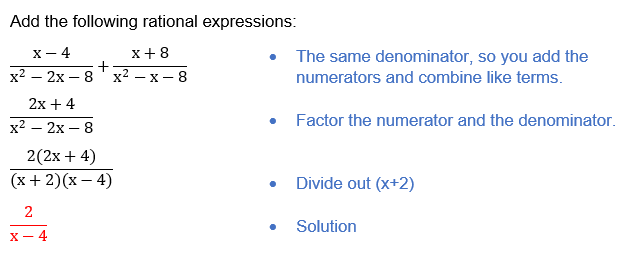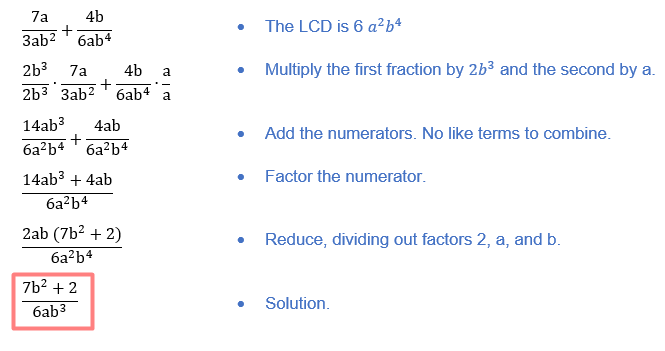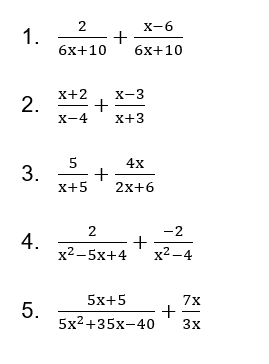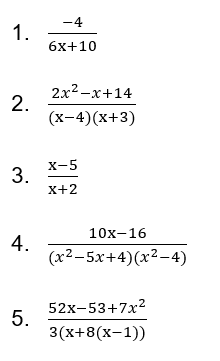A rational expression is one in which the denominator and numerator are both polynomials. By reducing the numerator and denominator to their simplest forms, rational expressions can be made simpler. The terms algebraic fraction and rational expression are interchangeable.

## What is the “Adding Rational Expressions Worksheet (with answer key + PDF)”?

This worksheet will explore some of the Adding Rational Expressions.

What is Rational Expression?

The ratio of two polynomials is an example of a rational expression. If an expression f is rational, it can be expressed in the form p/q, where p and q are polynomials. The fundamental arithmetic operations—addition, subtraction, multiplication, and division can be applied to rational expressions just like they can be applied to polynomials or any other kind of expression.

This worksheet will aid the student’s comprehension of the subject, particularly with regard to the addition of rational expressions.

Instructions on how to use the “Adding Rational Expressions Worksheet (with answer key + PDF).”

Study the concept and examples given and try to solve the given exercises below.

## Conclusion

The fact that any of these operations on two rational expressions always produces another rational expression is a nice feature of rational expressions. Contrary to polynomials, adding or subtracting two rational expressions is typically easier when they are multiplied or divided than when they are added or subtracted.

If you have any inquiries or feedback, please let us know.

A rational expression is an algebraic expression, for example, where both the numerator and the denominator are polynomials. There are values of x that are excluded from the rational expression because the denominator cannot be zero. The excluded value for the aforementioned expression is zero.

First, rewrite the fractions with a common denominator before adding rational expressions. We can directly add the numerators of two rational expressions if their denominators are already the same. To add two rational expressions generally:

• Find the LCD (LCM) of their denominators.
• Multiply and divide each rational expression by some expression/number such that all the denominators are the same as the LCD found in the above step.
• Now, we can add all the numerators as now the denominators are the same.

Example:Example:## Worksheet

1. Solve.1. Reflection

What do you think about solving the Adding Rational Expressions? Did you find it difficult to follow the pattern in each step?